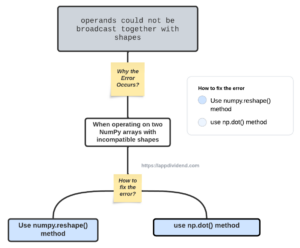# How to Fix ValueError: operands could not be broadcast together with shapesDiagram

To fix the ValueError: operands could not be broadcast together with shapes error in Python, use the “numpy.reshape()” method to ensure that the shapes of the arrays are compatible.

Python raises ValueError: operands could not be broadcast together with shapes error when operating on two NumPy arrays with incompatible shapes. Broadcasting refers to the ability of NumPy to handle arrays of different shapes during arithmetic operations.

## Solution 1

### Reproducing the error

``````import numpy as np

x = np.array([1, 2, 3, 4])
y = np.array([[1, 2], [3, 4]])

result = x + y
print(result)``````

Output

``ValueError: operands could not be broadcast together with shapes (3,) (2,2)``

In this example, array a has shape (3,), and array b has shape (2, 2). The shapes are incompatible for broadcasting, and the addition operation raises a ValueError.

### How to fix it?

Use the np.reshape() function to make both arrays compatible.

``````import numpy as np

x = np.array([1, 2, 3, 4])
y = np.array([[1, 2], [3, 4]])

x = x.reshape(2, 2)
result = x + y
print(result)``````

Output

``````[[2 4]
[6 8]]``````

We reshaped the x array to have the same shape as the y array and then performed the addition operation.

Since both x and y arrays have the same shape (2, 2), the addition operation can be performed element-wise, resulting in a new (2, 2) array with the summed values.

## Solution 2

The ValueError: operands could not be broadcast together with shapes also occurs when you try to perform matrix multiplication using a multiplication sign (*) in Python instead of the numpy.dot() function.

### Reproducing the error

``````import numpy as np

#define matrices
C = np.array([1, 2, 3, 4]).reshape(2, 2)
D = np.array([21, 19, 46, 52, 18, 23]).reshape(2, 3)

print(C*D)
``````

Output

``ValueError: operands could not be broadcast together with shapes (2,2) (2,3)``

### How to Fix it?

You can use the “np.dot()” method to fix the error.

``````import numpy as np

C = np.array([1, 2, 3, 4]).reshape(2, 2)
D = np.array([21, 19, 46, 52, 18, 23]).reshape(2, 3)

print(C.dot(D))
``````

Output

``````[[125 55 92]
[271 129 230]]
``````

That’s it.

This site uses Akismet to reduce spam. Learn how your comment data is processed.